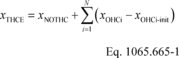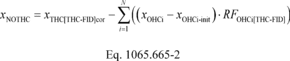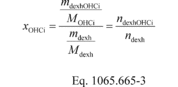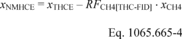# Title 40

## SECTION 1065.665

### 1065.665 THCE and NMHCE determination.

§ 1065.665 THCE and NMHCE determination.

(a) If you measured an oxygenated hydrocarbon's mass concentration, first calculate its molar concentration in the exhaust sample stream from which the sample was taken (raw or diluted exhaust), and convert this into a C1-equivalent molar concentration. Add these C1-equivalent molar concentrations to the molar concentration of non-oxygenated total hydrocarbon (NOTHC). The result is the molar concentration of total hydrocarbon equivalent (THCE). Calculate THCE concentration using the following equations, noting that Eq. 1065.665-3 is required only if you need to convert your oxygenated hydrocarbon (OHC) concentration from mass to moles:Where: xTHCE = the sum of the C1-equivalent concentrations of non-oxygenated hydrocarbon, alcohols, and aldehydes. xNOTHC = the sum of the C1-equivalent concentrations of NOTHC. xOHCi = the C1-equivalent concentration of oxygenated species i in diluted exhaust, not corrected for initial contamination. xOHCi-init = the C1-equivalent concentration of the initial system contamination (optional) of oxygenated species i, dry-to-wet corrected. xTHC[THC-FID]cor = the C1-equivalent response to NOTHC and all OHC in diluted exhaust, HC contamination and dry-to-wet corrected, as measured by the THC-FID. RFOHCi[THC-FID] = the response factor of the FID to species i relative to propane on a C1-equivalent basis. C# = the mean number of carbon atoms in the particular compound. Mdexh = the molar mass of diluted exhaust as determine in § 1065.340. mdexhOHCi = the mass of oxygenated species i in dilute exhaust. MOHCi = the C1-equivalent molecular weight of oxygenated species i. mdexh = the mass of diluted exhaust ndexhOHCi = the number of moles of oxygenated species i in total diluted exhaust flow. ndexh = the total diluted exhaust flow.

(b) If we require you to determine nonmethane hydrocarbon equivalent (NMHCE), use the following equation:Where: xNMHCE = the sum of the C1-equivalent concentrations of nonoxygenated nonmethane hydrocarbon (NONMHC), alcohols, and aldehydes. RFCH4[THC-FID] = the response factor of THC-FID to CH4. xCH4 = concentration of CH4, HC contamination (optional) and dry-to-wet corrected, as measured by the gas chromatograph FID.

(c) The following example shows how to determine NMHCE emissions based on ethanol (C2H5OH), methanol (CH3OH), acetaldehyde (C2H4O), and formaldehyde (CH2O) as C1-equivalent molar concentrations:

xTHC[THC-FID]cor = 145.6 µmol/mol xCH4 = 18.9 µmol/mol xC2H5OH = 100.8 µmol/mol xCH3OH = 1.1 µmol/mol xC2H4O = 19.1 µmol/mol xCH2O = 1.3 µmol/mol RFCH4[THC-FID] = 1.07 RFC2H5OH[THC-FID] = 0.76 RFCH3OH[THC-FID] = 0.74 RFH2H4O[THC-FID] = 0.50 RFCH2O[THC-FID] = 0.0 xNMHCE = xTHC[THC-FID]cor − (xC2H5OH · RFC2H5OH[THC-FID] + xCH3OH · RFCH3OH[THC-FID] + xC2H4O · RFC2H4O[THC-FID] + xCH2O · RFCH2O[THC-FID]) + xC2H5OH + xCH3OH + xC2H4O + xCH2O − (RFCH4[THC-FID] · xCH4) xNMHCE = 145.6 − (100.8 · 0.76 + 1.1 · 0.74 + 19.1 · 0.50 + 1.3 · 0) + 100.8 + 1.1 + 19.1 + 1.3 − (1.07 · 18.9) xNMHCE = 160.71 µmol/mol [79 FR 23805, Apr. 28, 2014, as amended at 81 FR 74187, Oct. 25, 2016]